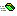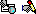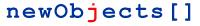HashCryptStreams RSA object

### Creation and ID-s

Program ID: newObjects.crypt.RSA
ProgramID: newObjects.crypt.RSA.free
ClassID: {92126712-F256-45bf-B3A4-2BDECD1EB78B}

### Overview

This object implements the raw RSA algorithm. Using it you can implement standard and custom applications of the algorithm.

### ReferenceCreateKeys Syntax: object.CreateKeys(n1,n2) Creates the RSA keys over the numbers specified. The object will seek for prime numbers P and Q starting with n1 for P and n2 for Q. This method is actually useful only if you want to use external random generator and pass starting numbers from it. The keys are generated in the properties: Modulus, SecretExp, PublicExp. Also P and Q are also available after calling this method. You can directly perform encryption/decryption after this call because it creates the all the required keys. See also DecryptPQ for more comments.CreateRandKeys Syntax: object.CreateRandKeys([size [,sizeexact]]) Creates the RSA keys using the internal random generator. The generated key is with Modulus long size + 1 bytes if sizeexact is omitted or False. If sizeexact is True the length of the Modulus is exactly size bytes. The size of the Modulus is actually the strength of the RSA encryption.  The method behaves this way to prevent the developers from mistakes when implementing generic encryption tasks. Generating slightly longer keys ensures that you will be able to encrypt size bytes with RSA. When implementing standards you should call the method with sizeexact set to True. Virtually all the standards (not only the official ones - such as PKCS) define certain transformations and limitations to the encrypted so that the amount of the data actually put through the RSA algorithm would be at least one byte shorter than the size of the keys.  The keys are generated in the properties: Modulus, SecretExp, PublicExp. Also P and Q are also available after calling this method. You can directly perform encryption/decryption after this call because it creates the all the required keys. See also DecryptPQ for more comments.Decrypt Syntax: result = object.Decrypt(data) Decrypts the passed data and returns it as binary data (byte array - variant holding VT_UI1 | VT_ARRAY). The data can be passed as binary data block or as hexdecimal string. In order this method to work you need to set first Modulus and SecretExp.DecryptPQ Syntax: result = object.DecryptPQ(data) Decrypts the passed data and returns it as binary data (byte array - variant holding VT_UI1 | VT_ARRAY). The data can be passed as binary data block or as hexdecimal string. In order this method to work you need to set first P, Q, Modulus and SecretExp.Encrypt Syntax: result = object.Encrypt(data) Encrypts the passed data and returns the result as binary data (byte array - variant holding VT_UI1 | VT_ARRAY). The data can be passed as binary data block or as hexdecimal string. In order this method to work you need to set first Modulus and PublicExp.Reset Syntax: object.Reset Resets the object clearing P, Q, Modulus, SecretExp, PublicExp.Modulus Syntax: object.Modulus = v v = object.Modulus Puts/Gets the Modulus (P * Q).P Syntax: object.P = v v = object.P Puts/Gets P - the first prime number.Q Syntax: object.Q = v v = object.Q Puts/Gets Q - the second prime number.PublicExp Syntax: object.PublicExp = v v = object.PublicExp Puts/Gets the public exponent (the public part of the key).SecretExp Syntax: object.SecretExp = v v = object.SecretExp Puts/Gets the private exponent (the private part of the key)

...Copyright 2001-2006 newObjects [ ]